Courses

# Frequency Domain Analysis of Control Systems - 1

## 10 Questions MCQ Test Topicwise Question Bank for Electronics Engineering | Frequency Domain Analysis of Control Systems - 1

Description
This mock test of Frequency Domain Analysis of Control Systems - 1 for Electrical Engineering (EE) helps you for every Electrical Engineering (EE) entrance exam. This contains 10 Multiple Choice Questions for Electrical Engineering (EE) Frequency Domain Analysis of Control Systems - 1 (mcq) to study with solutions a complete question bank. The solved questions answers in this Frequency Domain Analysis of Control Systems - 1 quiz give you a good mix of easy questions and tough questions. Electrical Engineering (EE) students definitely take this Frequency Domain Analysis of Control Systems - 1 exercise for a better result in the exam. You can find other Frequency Domain Analysis of Control Systems - 1 extra questions, long questions & short questions for Electrical Engineering (EE) on EduRev as well by searching above.
QUESTION: 1

Solution:
QUESTION: 2

Solution:
QUESTION: 3

### The initial slope of Bode plot for a transfer function having no poles at origin is

Solution:

With no-pole at origin, initial slope will be OdB/decade.
For n-pole at origin, initial slope will be -20 ndB/decade.

QUESTION: 4

The gain margin of a control system having the loop transfer function G(s)H(s) =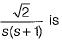Solution: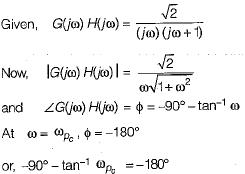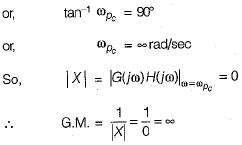QUESTION: 5

Gain crossover frequency is the frequency at which the gain of G(jω) is

Solution: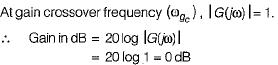QUESTION: 6

For a control system having gain margin of -10 dB, the magnitude of GH(s) for 180° phase shift is

Solution: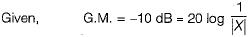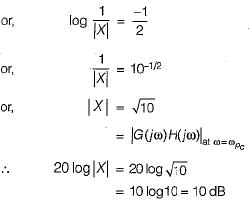QUESTION: 7

If the Nyquist plot of the loop transfer function G(s)H(s) of a closed-loop system does not encloses the (-1, j0) point in the G(s)H(s) plane, the gain margin of the system will be

Solution:

Since the critical point (-1 + j0 ) is not enclosed, therefore m agnitude of OLTF,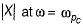will be less than unity.
Therefore, G.M. = 20 log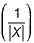will be positive and the system will be stable.

QUESTION: 8

A system with phase margin close to zero or gain margin close to unity is

Solution:
QUESTION: 9

If the transfer function of a first-order system in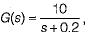then the time constant of this system will be

Solution: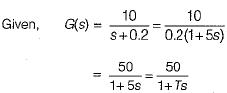Hence, time constant = T= 5 seconds

QUESTION: 10

The polar plot of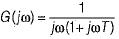Solution: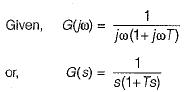Given system is of type-1 and order-2, therefore its polar plot will be as shown below.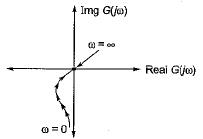Hence, the polar plot will neither cross the real axis nor the imaginary axis.